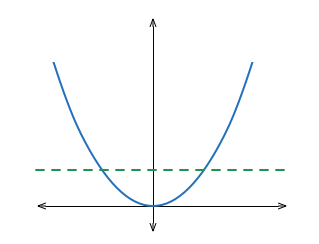Probability

# 1-dimensional Geometric ProbabilityA real number $r$ is chosen at random from the interval $[ -1 , 1 ]$.

What is the probability that $r ^ 2< \frac{1}{9}$?

A real number is chosen at random from the interval $[ -5, 12 ]$. What is the probability that the real number is less than $1$?

A point is chosen uniformly at random on the real line, in the interval $(0, 1)$. What is the probability that the chosen point is closer to the point $0$ than it is to the point $0.38$?

Two integers are randomly and independently chosen from $13$ to $22$ (inclusive). Now, Kevin picks an integer from $13$ to $22$ wanting the probability that his number is not less than either of two to be more than $50$%. What is the least number he can pick?

Details and assumptions

The two numbers randomly and independently chosen could be the same.

A point is chosen at random from the unit interval $[0, 1]$. What is the probability that it is closer to $0.66$ than it is to $0.28$?

×